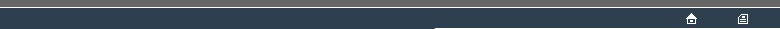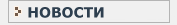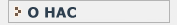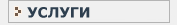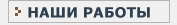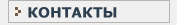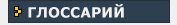СТАТИСТИЧЕСКИЙ В данном разделе Вы найдете все интересующие Вас термины и определения, связанные с статистикой и статистическими исследованиями.КЛИНИЧЕСКИЕ ИССЛЕДОВАНИЯ Необходимый перечень терминов и определений, используемых при проведении клинических испытанийW WILCOXON RANK-SUM TEST See : WILCOXON TEST(1), WILCOXON TEST(2). WILCOXON TEST(1) [Named after the statistician F, Wilcoxon ()] This test applies to an EXPERIMENTAL DESIGN involving two REPEATED MEASURE observations on a common set of experimental units, which need be only ORDINAL-SCALE. The purpose is to measure shift in scale location between the two levels of the REPEATED MEASURE distinction. The TEST STATISTIC is derived from the set of differences between the two levels of the REPEATED MEASURE distinction - one difference score for each observational unit. The procedure is somewhat variable between authors, although the variants each correspond to valid well-sized EXACT TEST(1)s. Wilcoxon's original procedure commences by discarding entirely the observations from any experimental units for which the data values are equal at each level of the REPEATED MEASURE comparison. Thus or otherwise, the next step is RANKING the differences, providing a rank for each retained experimental unit; the ranks are according to the absolute values of the differences. The ranks are summed separately into two or three categories : negative differences; zero differences (if any); positive differences. The TEST STATISTIC is the smaller of the outer categories, plus an adjustment for the middle (zero-difference) category. Also see : PITMAN PERMUTATION TEST(2). WILCOXON TEST(2) [Named after the statistician F, Wilcoxon ()] This is a test for an EXPERIMENTAL DESIGN involving two INDEPENDENT GROUPS of experimental units, where data need be only ORDINAL-SCALE. The purpose is to measure shift in scale location between the two groups. The TEST STATISTIC is the sum, for a nominated group, of the ranks of the data for the groups combined. This test has an EQUIVALENT TEST STATISTIC to that for the MANN-WHITNEY TEST, so the two tests must always agree. Also see : PITMAN PERMUTATION TEST(1). 2-WAY TABLE See : TWO-WAY TABLE. 2-BY-2 TABLE This is a TWO-WAY TABLE where the numbers of levels of the row- and column-classifications are each 2. If the row- and column- classifications each divide the observational units into subsets, then it is likely that it will be useful to analyse the data using the FISHER TEST(1).W
WILCOXON RANK-SUM TEST
WILCOXON TEST(1)
WILCOXON TEST(2)
2-WAY TABLE
2-BY-2 TABLEНовости   О нас   Услуги   Наши работы   Статьи   Контакты   Глоссарий
Статистическая помощь! © 2005 - 2023 • Защита авторских прав